Next: Worked Examples Up: Magnetism Previous: Gauss' Law for Magnetic

## Galvanometers

We have talked a lot about potential differences, currents, and resistances, but we have not talked much about how these quantities can be measured. Let us now investigate this topic.

Broadly speaking, only electric currents can be measured directly. Potential differences and resistances are usually inferred from measurements of electric currents. The most accurate method of measuring an electric current is by using a device called a galvanometer.

A galvanometer consists of a rectangular conducting coil which is free to pivot vertically in an approximately uniform horizontal magnetic field--see Fig. 31. The magnetic field is usually generated by a permanent magnet. Suppose that a currentruns through the coil. What are the forces exerted on the coil by the magnetic field? According to Eq. (152), the forces exerted on those sections of the coil in which the current runs in the horizontal plane are directed vertically up or down. These forces are irrelevant, since they are absorbed by the support structure of the coil, which does not allow the coil to move vertically. Equation (152) also implies that the force exerted on the section of the coil in which the current flows downward is of magnitude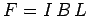, where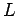the length of this section, and is directed out of the page (in the figure). Likewise, the force exerted on the section of the coil in which the current flows upward is also of magnitude, and is directed into the page. These two forces exert a torque on the coil which tries to twist it about its vertical axis in an anti-clockwise direction (looking from above). Using the usual definition of torque (i.e., torque is the product of the force and the perpendicular distance from the line of action of the force to the axis of rotation), the net torque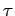acting on the coil is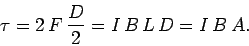(183)

where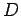is the horizontal width of the coil, andis its area. Note that the two vertical sections of the coil give rise to equal contributions to the torque. Strictly speaking, the above expression is only valid when the coil lies in the plane of the magnetic field. However, galvanometers are usually constructed with curved magnetic pole pieces in order to ensure that, as the coil turns, it always remains in the plane of the magnetic field. It follows that, for fixed magnetic field-strength, and fixed coil area, the torque exerted on the coil is directly proportional to the current.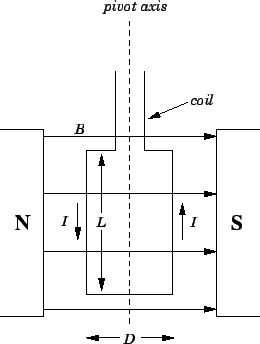The coil in a galvanometer is usually suspended from a torsion wire. The wire exerts a restoring torque on the coil which tries to twist it back to its original position. The strength of this restoring torque is directly proportional to the angle of twist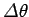. It follows that, in equilibrium, where the magnetic torque balances the restoring torque, the angle of twistis directly proportional to the currentflowing around the coil. The angle of twist can be measured by attaching a pointer to the coil, or, even better, by mounting a mirror on the coil, and reflecting a light beam off the mirror. Since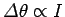, the device can easily be calibrated by running a known current through it.

There is, of course, a practical limit to how large the angle of twistcan become in a galvanometer. If the torsion wire is twisted through too great an angle then it will deform permanently, and will eventually snap. In order to prevent this from happening, most galvanometers are equipped with a stop'' which physically prevents the coil from twisting through more than (say)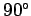. Thus, there is a maximum current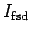which a galvanometer can measure. This is usually referred to as the full-scale-deflection current. The full-scale-deflection current in conventional galvanometers is usually pretty small: e.g.,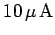. So, what do we do if we want to measure a large current?

What we do is to connect a shunt resistor in parallel with the galvanometer, so that most of the current flows through the resistor, and only a small fraction of the current flows through the galvanometer itself. This is illustrated in Fig. 32. Let the resistance of the galvanometer be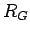, and the resistance of the shunt resistor be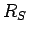. Suppose that we want to be able to measure the total currentflowing through the galvanometer and the shunt resistor up to a maximum value of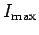. We can achieve this if the current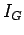flowing through the galvanometer equals the full-scale-deflection currentwhen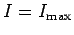. In this case, the current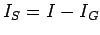flowing through the shunt resistor takes the value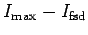. The potential drop across the shunt resistor is therefore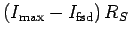. This potential drop must match the potential drop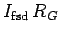across the galvanometer, since the galvanometer is connected in parallel with the shunt resistor. It follows that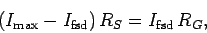(184)

which reduces to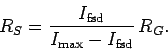(185)

Using this formula, we can always choose an appropriate shunt resistor to allow a galvanometer to measure any current, no matter how large. For instance, if the full-scale-deflection current is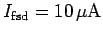, the maximum current we wish to measure is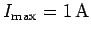, and the resistance of the galvanometer is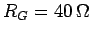, then the appropriate shunt resistance is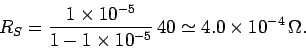(186)

Most galvanometers are equipped with a dial which allows us to choose between various alternative ranges of currents which the device can measure: e.g., 0-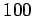mA, 0-1A, or 0-10A. All the dial does is to switch between different shunt resistors connected in parallel with the galvanometer itself. Note, finally, that the equivalent resistance of the galvanometer and its shunt resistor is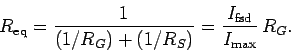(187)

Clearly, if the full-scale-deflection currentis much less than the maximum currentwhich we wish to measure then the equivalent resistance is very small indeed. Thus, there is an advantage to making the full-scale-deflection current of a galvanometer small. A small full-scale-deflection current implies a small equivalent resistance of the galvanometer, which means that the galvanometer can be connected into a circuit without seriously disturbing the currents flowing around that circuit.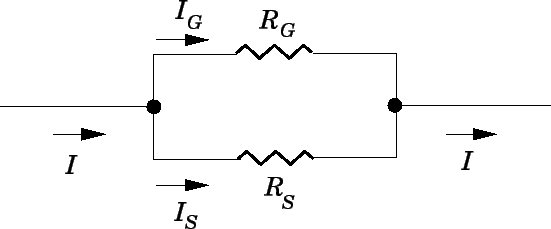A galvanometer can be used to measure potential difference as well as current (although, in the former case, it is really measuring current). In order to measure the potential differencebetween two pointsand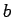in some circuit, we connect a galvanometer, in series with a shunt resistor, across these two points--see Fig. 33. The galvanometer draws a currentfrom the circuit. This current is, of course, proportional to the potential difference betweenand, which enables us to relate the reading on the galvanometer to the voltage we are trying to measure. Suppose that we wish to measure voltages in the range 0 to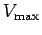. What is an appropriate choice of the shunt resistance? Well, the equivalent resistance of the shunt resistor and the galvanometer is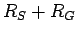, whereis the resistance of the galvanometer. Thus, the current flowing through the galvanometer is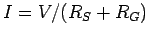. We want this current to equal the full-scale-deflection currentof the galvanometer when the potential difference between pointsandattains its maximum allowed value. It follows that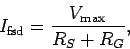(188)

which yields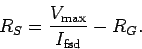(189)

Using this formula, we can always choose an appropriate shunt resistor to allow a galvanometer to measure any voltage, no matter how large. For instance, if the full-scale-deflection current is, the maximum voltage we wish to measure is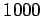V, and the resistance of the galvanometer is, then the appropriate shunt resistance is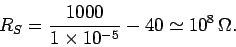(190)

Again, there is an advantage in making the full-scale-deflection current of a galvanometer used as a voltmeter small, because, when it is properly set up, the galvanometer never draws more current from the circuit than its full-scale-deflection current. If this current is small then the galvanometer can measure potential differences in a circuit without significantly perturbing the currents flowing around that circuit.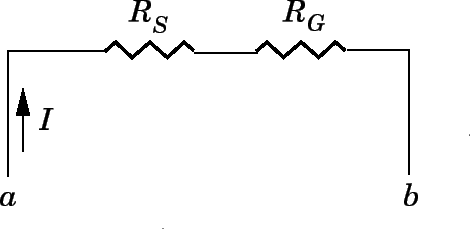Next: Worked Examples Up: Magnetism Previous: Gauss' Law for Magnetic
Richard Fitzpatrick 2007-07-14